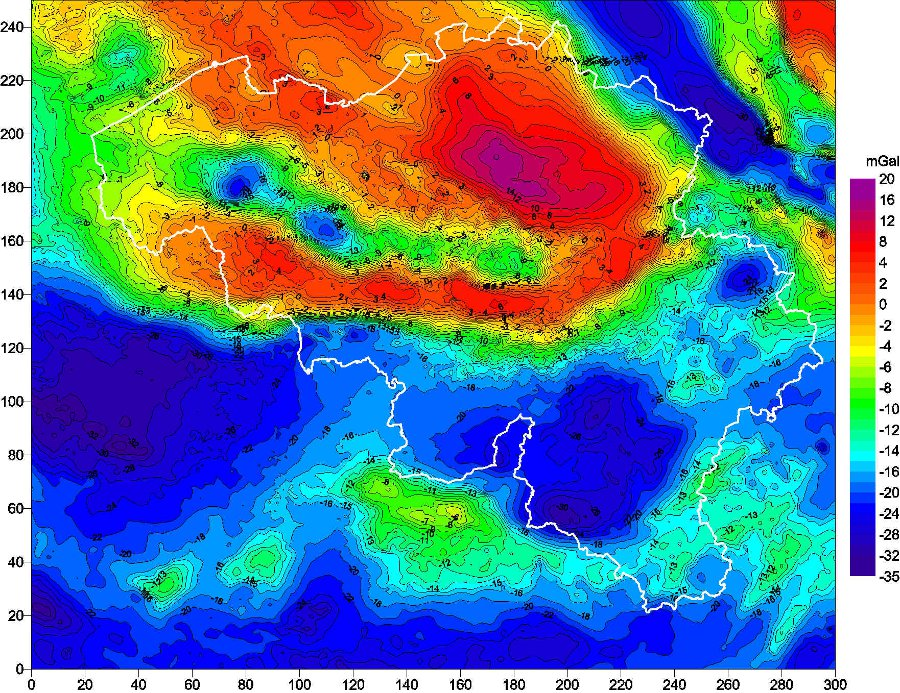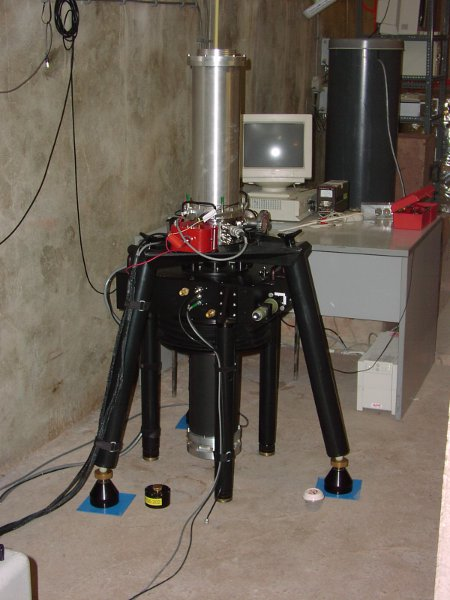The gravity force, which allows us to define the weight of an object, keeps us at the Earth’s surface and is the origin of the free fall of a body. This force is proportional with the mass : $\vec{F} = m \vec{g}$ , where $\vec{g}$ is the gravity acceleration. The magnitude of this acceleration is the gravity g, which is measured with a gravimeter. This is a physical quantity varying in time and space. Indeed, g depends, mainly, on the latitude, the mass distribution in the Earth’s interior, the Earth’s rotation (velocity and position of the rotation axis), and the relative positions of the Moon and the Sun, which cause the tides.

The relative or absolute determination of g is essential in several areas of the scientific research :

• In geophysics, one measures the gravity variation to study tectonic deformations, the post-glacial rebound, the tides, the influence of the atmosphere and the hydrosphere, and the structure of the globe from the inner core to the Earth’s crust. On the other hand, the analysis of the local variations of g presents numerous applications in geology.Figure : Gravimetric anomalies (Bouguer, in mGal [1 mGal $\Leftrightarrow$ 10 µm/s2 $\Leftrightarrow$ 0.001 g]) in our regions. This map reflects the variations in density of the underground rocks. It allows a visualization of large regional geologic structures. The Brabant Massive, composed of denser rocks, is the red part in the northeast of the map. The blue part corresponds to the influence of the less dense rocks in the Ardenne and the Paris Basin. The Roer Graben, in blue in the northeast, consists in an accumulation of less dense and more recent sediments.
• In metrology, g is to play a role in the new realization of the kilogram.
• Finally, g is indispensable to geodesy, for the determination of the geoid and therefore of the heights (the geoid represents the average level of the seas and their prolongation under the continents).

The Observatory has acquired an international reputation in all these fields in terms of scientific knowledge as well as technical expertise. For this purpose, the Observatory has relative spring gravimeters, a relative superconducting gravimeter and an absolute gravimeter.

In a relative gravimeter, the displacement of a suspended mass is proportional to the variations of g. In most of the cases it concerns mobile instruments dedicated to field measurements. Besides these gravimeters, which only measure small variations of g, the Observatory owns an absolute gravimeter since 1996, which gives the real value of g with an accuracy of one billionth (10-9) of g and allows a precise control of the relative gravimeters. In the absolute gravimeter, a test mass is repeatedly dropped in a vacuum chamber and its position is measured as a function of time, which provides the gravity value g.

The calibration factor of relative spring gravimeters often suffers from time variations and their measurements present a strong time drift too. Therefore, the Observatory installed a superconducting gravimeter in 1995 at the Membach station, near Eupen, and another one in Rochefort end 2014. In such an instrument, the spring suspension is replaced by the magnetic levitation of a superconducting sphere, generated by constant currents trapped in two superconducting coils. The sphere and the coils are temperature regulated to –269°C and this provides a highly sensitive gravimeter that is stable for long periods. The superconducting gravimeter measures g variations with a precision a hundred times better than the spring instruments. In contrast with spring gravimeters, the superconducting gravimeter is not mobile, however.

The measurements of the superconducting and absolute gravimeters allow the study of long-term phenomena, such as a slow ground deformation or slow changes in the ground water content. As high accuracy reference instrument, the absolute gravimeter also participates in several international comparisons and provides the base points of the Belgian gravimetric network, surveyed with spring gravimeters.Figures : The absolute gravimeter FG5#202 determines the gravity in Membach with an accuracy of 10-9 g. As a function of the seasons, the value of g varies from 9,810 467 20 to 9,810 470 30 m/s2, after correction of the known variable effects such as the tides. Since 1995, the superconducting gravimeter GWR#C021 measures continuously the variations of the gravity in Membach with a precision better than 10-10 g. The spring gravimeter Scintrex CG5 is a field instrument, which measures the variations of the gravity with a precision of 10-8 g.